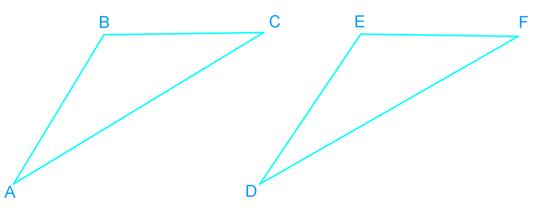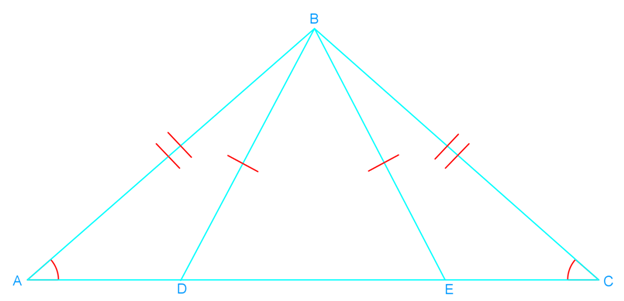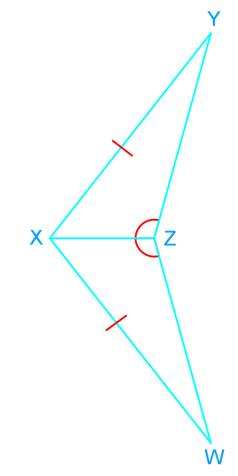# Triangles congruent by ASA and AAS proofs

### Triangles congruent by ASA and AAS proofs

#### Lessons

• Introduction
a)
1. Similar tirangles VS. Congruent triangles
2. Ways to prove congruency:
• SSS
• SAS
• ASA
• AAS

• 1.
What is the third congruence that is needed to prove that $\triangle ABC$$\cong$$\triangle DEF$ using the given postulate or theorem?a)
$\overline {AB}$$\cong$$\overline {DE}$ and $\angle C$$\cong$$\angle F$
Prove by AAS.

b)
$\overline {AC}$$\cong$$\overline {DF}$ and $\angle A$$\cong$$\angle D$
Prove by ASA.

c)
$\angle A$$\cong$$\angle D$ and $\angle B$$\cong$$\angle E$
Prove by AAS.

• 2.
Decide if the following triangles are congruent. Show your reasoning.
a)
$\triangle ABC$, $\triangle CBD$b)
$\triangle XYZ$, $\triangle XWZ$